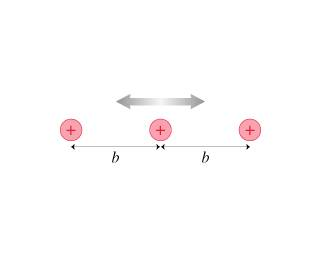# Four lowest vibrational states of these ions

• Archived
albertsmith

## Homework StatementIn most metals, the atomic ions form a regular arrangement called a crystal lattice. The conduction electrons in the sea of electrons move through this lattice. The figure (Intro 1 figure) is a one-dimensional model of a crystal lattice. The ions have mass m , charge e , and an equilibrium separation b.

## Homework Equations

Suppose this crystal consists of aluminum ions with an equilibrium spacing of 0.30nm. What are the energies of the four lowest vibrational states of these ions?

## The Attempt at a Solution

well i thought separation equilibrium = (h bar/mw)^0.5 from this i got the w angular frequency and then i use the vibrational formula (n-1)h bar * w i got these first four vibrational states 0.42ev,1.30ev,2,1ev,2,9ev but i got it wrong can anybody tell what i did wrong please thanks